# QtiPlot - Data Analysis and Scientific Visualisation

## Descriptive Statistics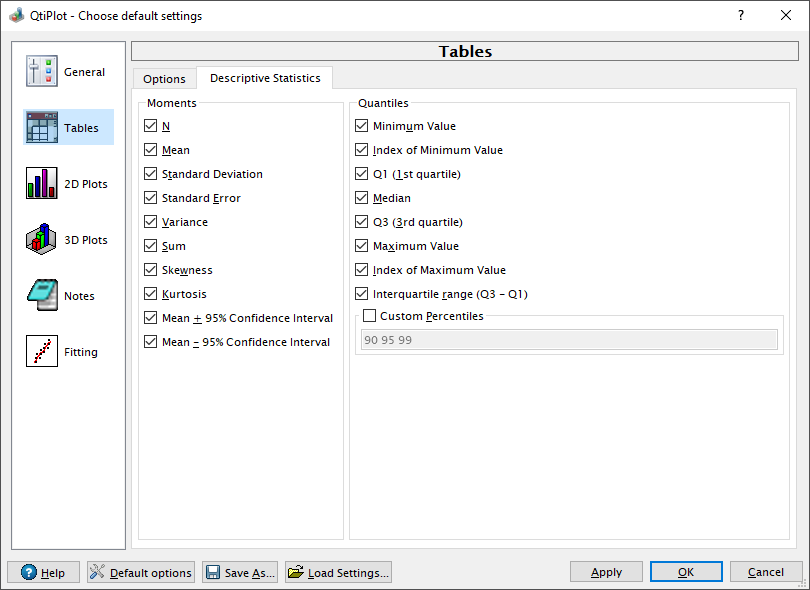The following moments and quantiles can be computed by QtiPlot on table columns or rows: mean, standard deviation, standard error, variance, sum, skewness, kurtosis, minimum and maximum values, median, first and third quantile and the interquartile range.

It is also possible to define a list of custom percentiles values between 1 and 99 that can be calculated on table columns/rows.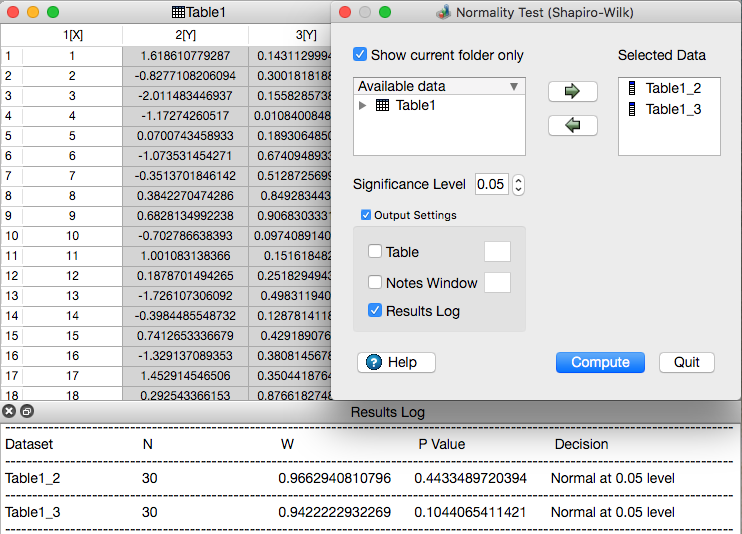QtiPlot provides a frequency count dialog that can be used in order to calculate the frequency distribution of the data values from a table column.

The normality test (Shapiro-Wilk method) can be used to verify the null hypothesis that a data sample came from a normally distributed population.

## Parametric Tests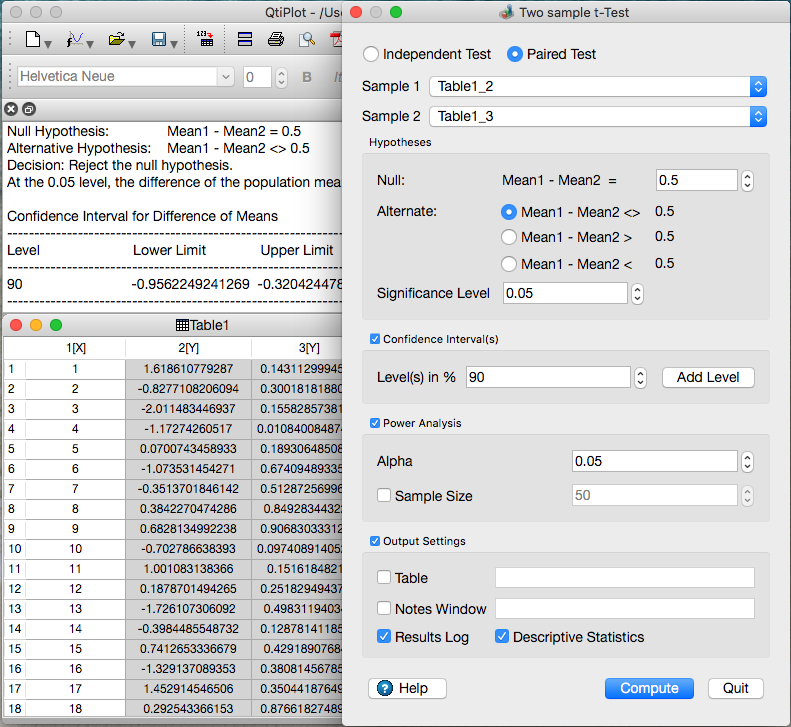A t-test is any statistical hypothesis test in which the test statistic follows a Student's t-distribution under the null hypothesis.

QtiPlot can perform several forms of the parametric Student's t-test: one sample, two sample and paired sample t-test.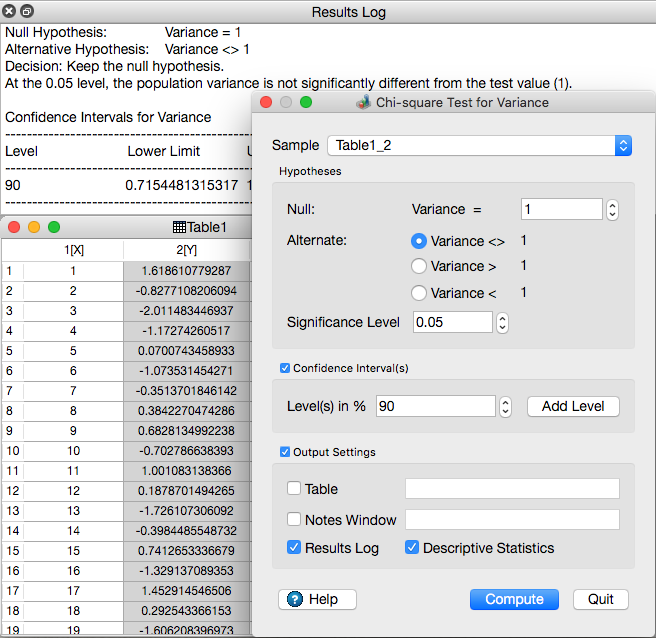A chi-squared test is any statistical hypothesis test where the sampling distribution of the test statistic is a chi-squared distribution when the null hypothesis is true. QtiPlot can perform the chi-square test for variance.

## Non-parametric Tests

QtiPlot can perform the following non-parametric tests: Mann-Whitney, Kolmogorov-Smirnov, Wilcoxon signed rank test (one sample and paired sample), the paired sample sign test, Mood's median test, Kruskal-Wallis ANOVA and Friedman ANOVA.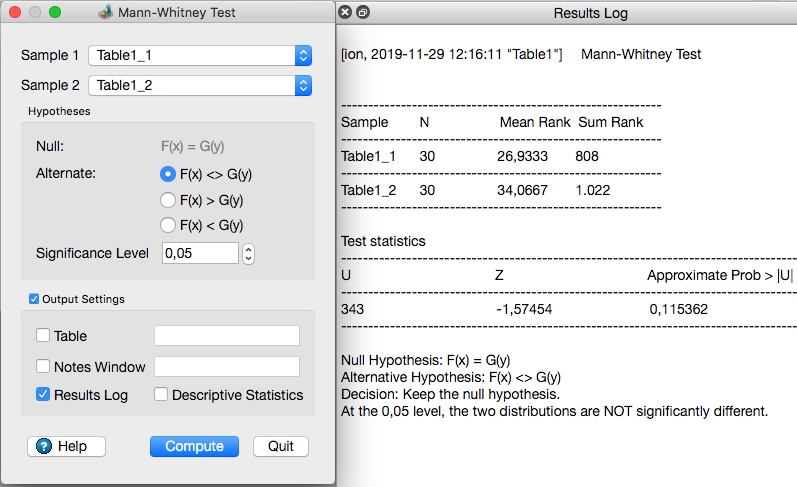The Mann-Whitney test can be used in order to determine whether two independent samples were selected from populations having the same distribution.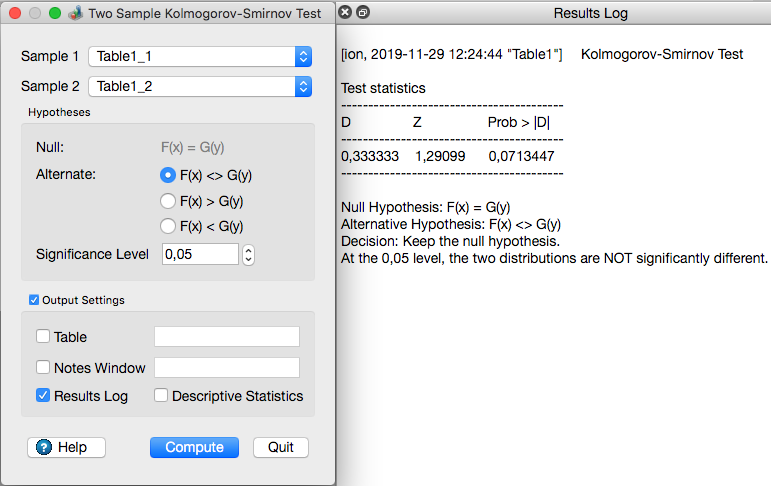The Kolmogorov-Smirnov test can be used in order to estimate the statistical likelihood that two samples are drawn from the same distribution.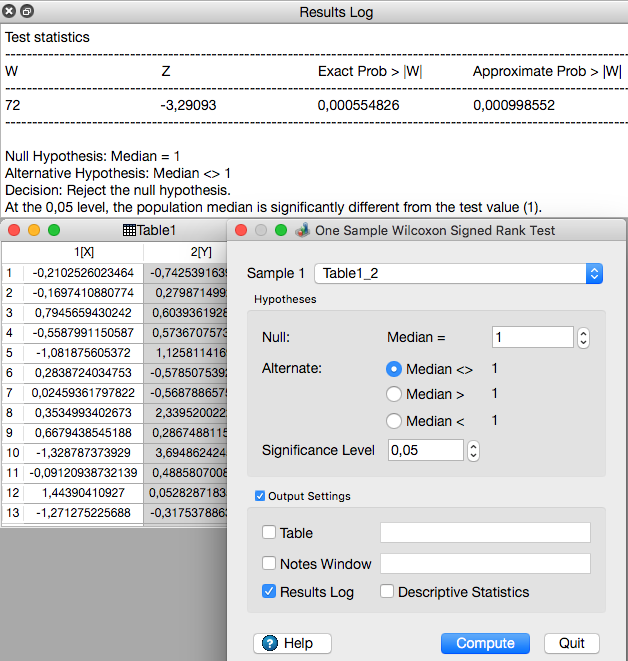The one sample Wilcoxon signed rank test can be used as a non-parametric alternative to a one sample t-test.

This test determines whether the median of the data sample is equal to a specific value.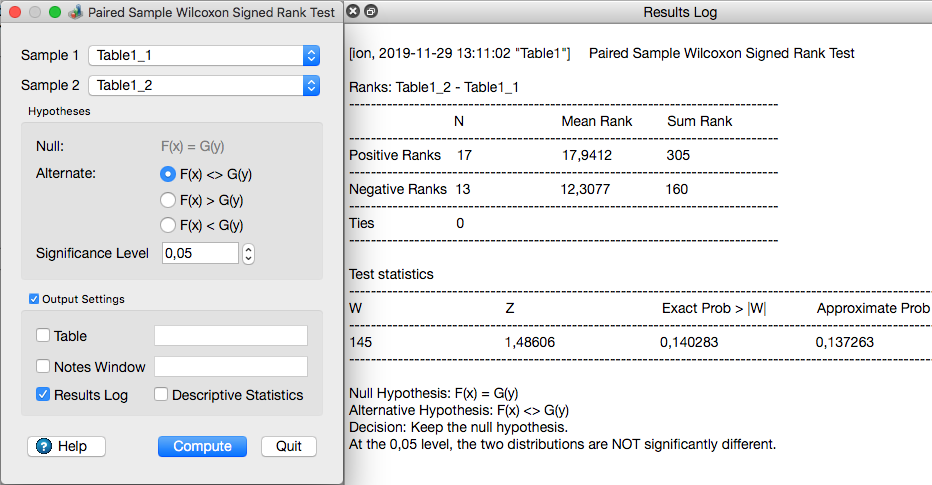The paired sample Wilcoxon signed rank test can be used in order to determine whether two dependent samples were selected from populations having the same distribution.

It can be use as an alternative to the paired sample Student's t-test.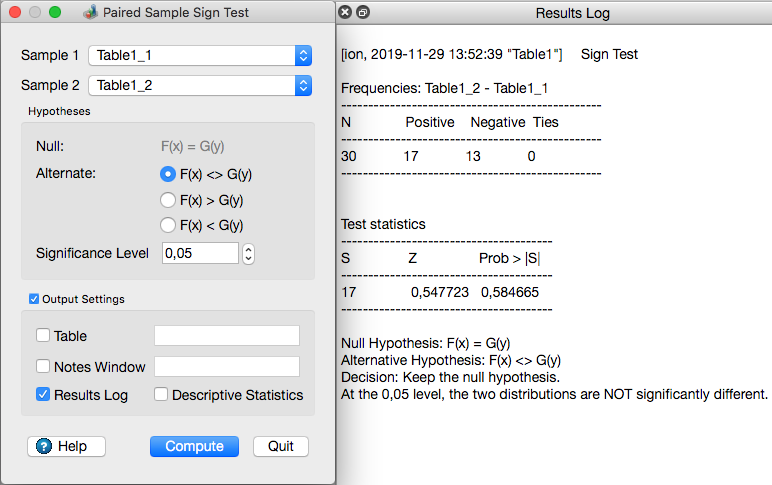The paired sample sign test is a statistical method that can be used in order to investigate for consistent differences between pairs of observations.

QtiPlot computes the test statistics and a probability using the cumulative distribution function of the binomial distribution. The null hypothesis is rejected if the probability is lower than the value of the user defined significance level.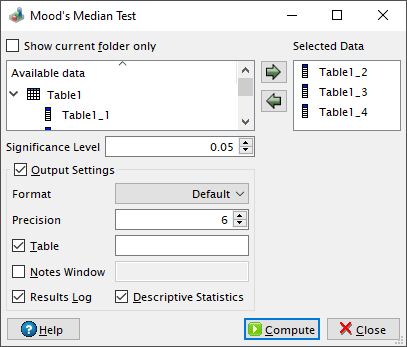Mood's median test can be used in order to test whether several samples originate from the same distribution.

This test uses the chi-square statistic (χ2). QtiPlot computes an approximated p-value, which is the probability of a chi-squared value of at least χ2 being observed, using the lower tail of the cumulative distribution function for the chi-squared distribution. The null hypothesis is rejected if the approximated probability is lower than the value of input significance level.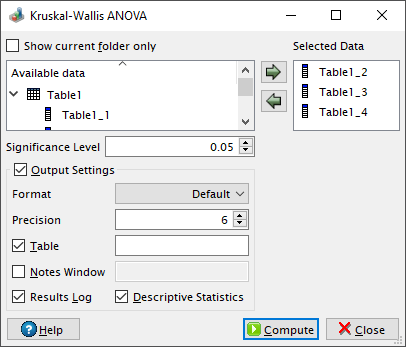Kruskal-Wallis ANOVA can be used in order to test whether several samples originate from the same distribution.

QtiPlot computes the test statistic (H) and an approximated p-value. The approximation is based on the fact that when the size of the input samples (Ni) or the number of samples (k) is large (i.e. Ni > 5 or k ≥ 3), the probability distribution of H is that of a chi-squared distribution whith k-1 degrees of freedom. The p-value computed by QtiPlot is the probability of a chi-squared value of at least H being observed. The null hypothesis is rejected if the approximated probability is lower than the value of the significance level.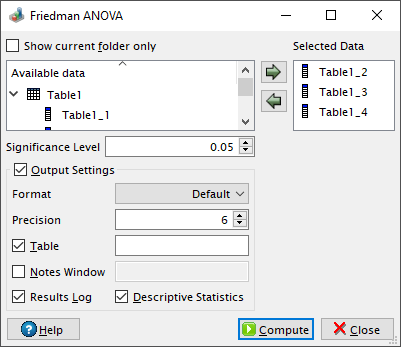Friedman ANOVA is a non-parametric statistical test developed by Milton Friedman that can be used in order to test whether several samples originate from the same distribution.

QtiPlot computes the test statistic (Q) and an approximated p-value. The approximation is based on the fact that when the size of the input samples (N) or the number of samples (k) is large (i.e. N > 15 or k > 4), the probability distribution of Q is that of a chi-squared distribution whith k-1 degrees of freedom. The p-value computed by QtiPlot is the probability of a chi-squared value of at least Q being observed. The null hypothesis is rejected if the approximated probability is lower than the value of the significance level.

## ANOVAThe one-way analysis of variance (abbreviated one-way ANOVA) can be used to compare the means of two or more samples using the F distribution.

QtiPlot can perform several post-hoc analysis tests that can determine which level means or sample means are significantly different from each other. The folowing post-hoc tests are currently implemented: Tukey, Bonferroni, Dunn-Sidak, Fisher's Least Significant Difference (LSD), Scheffe, Holm-Bonferroni and Holm-Sidak.The two-way analysis of variance (abbreviated two-way ANOVA) can be used to compare the means of two or more samples using the F distribution.

In the QtiPlot implementation of two-way ANOVA there are two factors defined, A and B, with LA and LB levels respectively, for a total number of samples N = LALB.

QtiPlot can perform several post-hoc analysis tests that can determine which level means or sample means are significantly different from each other. The folowing post-hoc tests are currently implemented: Tukey, Bonferroni, Dunn-Sidak, Fisher's Least Significant Difference (LSD), Scheffe, Holm-Bonferroni and Holm-Sidak.